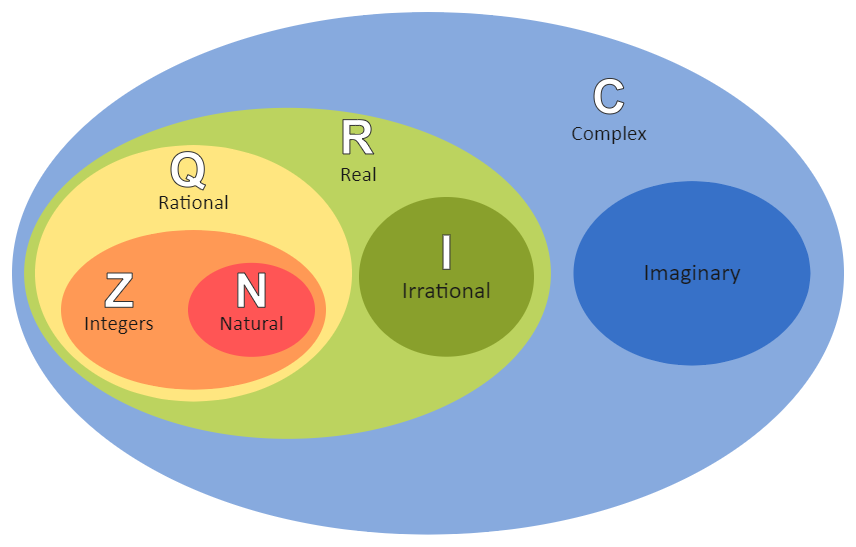Template Community / Rational Number Venn Diagram

# Rational Number Venn DiagramBlackwood
Published on 2022-04-20
Edit OnlineStudents can use this Rational Numbers Venn Diagram to classify natural/counting, whole, integers, rational, and irrational numbers. Numbers can be cut and pasted into the diagram or typed in. The rational number is also highlighted in this Rational Number Venn Diagram. A rational number in arithmetic is a number that can be represented as the quotient p/q of two integers such that q = 0. Aside from fractions, all integers are included in the set of rational numbers. Each can be expressed as a quotient, with the integer and one as the denominators. Rational numbers are real numbers that can be defined as a ratio of two integers, such as P/Q, where Q is greater than zero.
Tag
venn diagram
Report
0
116PostRecommended Templates